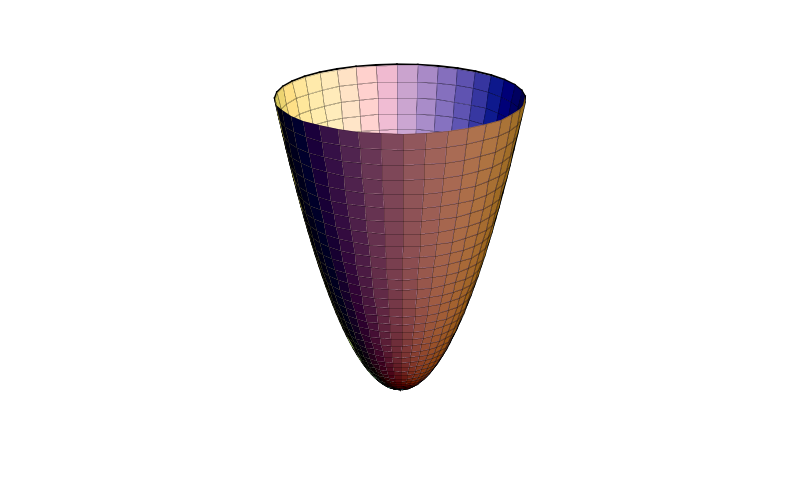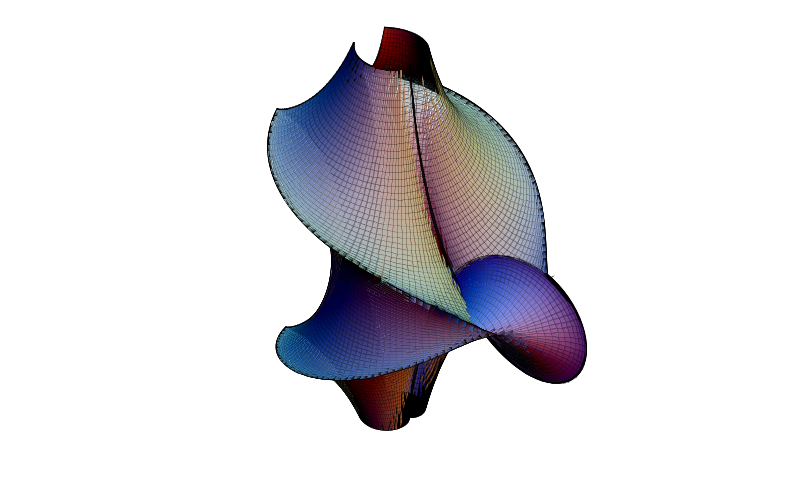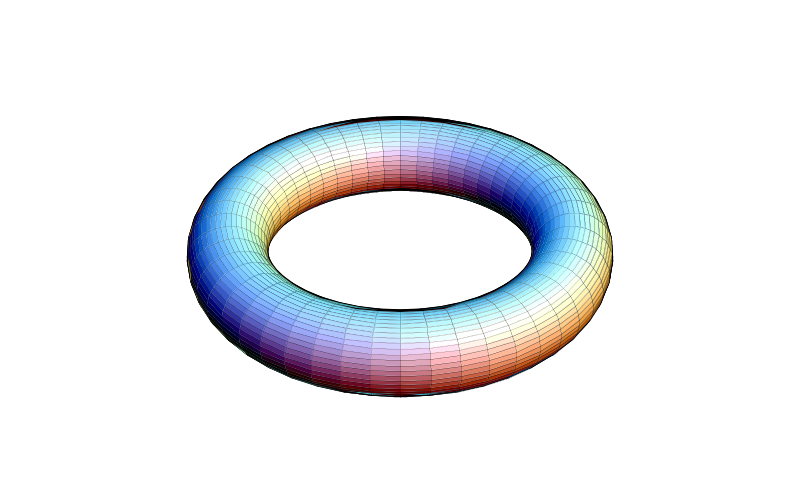# DMelt:Plots/6 Math Exhibits

Limitted access. First login to DataMelt if you are a full DataMelt member. Then login to HandWiki as a user.

# 3D math exhibits

In addition to the HPlot, HPlot2D, HPlot3D canvaces, DataMelt offers another canvas for for visualizing and experimenting with a variety of mathematical objects or "exhibits." This class is calledjhplot.HPlotMX

The class is based on 3D-XplorMath program of the 3DXM Consortium. DataMelt uses the base code of this program but adds a support for vector graphics i.e. one can export images to EPS, SVG or PDF formats. Thus, all images are fully scalable and are ready to be included to publications. If you need a similar program but with animation support (but without vector graphics export), use the DataMelt IDE ("See the Plot menu).

Here is a simple Jython script which creates a predefined paraboloid:

from jhplot import *
c1=HPlotMX("vmm3d.surface.parametric.Paraboloid")
c1.visible()

Here we simply call the class name that defines "Paraboloid". The output of this script is shown below:A similar example can be rewritten in BeanShell, Groovy or Java languages. Here is the same example in the Groovy scripting. Make a file "test.groovy", and run it in DataMelt:

import jhplot.*
c1=new HPlotMX("vmm3d.surface.parametric.Paraboloid")
c1.visible()

In the above example we simply call the class name that defines a paraboloid function, seevmm3d.surface.parametric.Paraboloid class. There are about 60 predefined parametric mathematical functions that can be called to display. They are listed in the description of parametric functions in thevmm3d/surface/parametric/package-summary.

From the above list, one can pick up any math object and path its name asjava.lang.String. Alternatively, one can construct mathematical exhibit by creating the object itself:

The output is mathematical object called "Kuen Surface" shown below:As usual, use the method "export" to create an image file. For example, we can create EPS, PDF and SVG figures using the vector formats as:

c1.export("KuenSurface.eps")
c1.export("KuenSurface.pdf")
c1.export("KuenSurface.svg")

## Showing custom functions

Now we consider how to draw custom parametric or non-parametric functions, instead of showing predefined functions. First we create a function of torus using pure-Java approach:

### Using Java to build custom function

Here is an example showing how to create a 3D surface plot using Java programming language:

The defined torus will be shown below:### Using Jython to build custom function

Instead of defining the function as a Java code, one can define it inside the same Jython script that is used to show the canvas with the object. Here is a simple Jython code create torus in 3D:

Run this Jython/Python script and you will see the identical torus. You can change any parameters. For example, inserting "setV(0,2)", we will generate only a part of the torus as shown bellow:You can reduce the number of patches used to draw the torus, making this picture rendering faster.

### Using Groovy to build custom function

Here is the same method to create a torus using Groovy scripting: